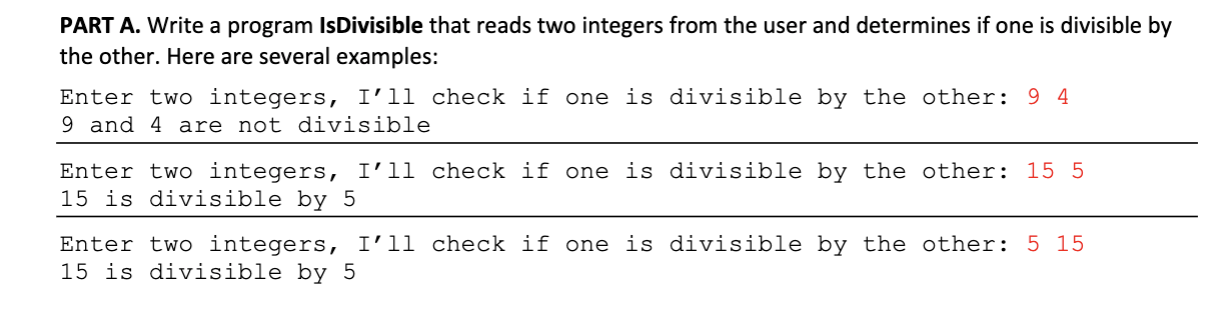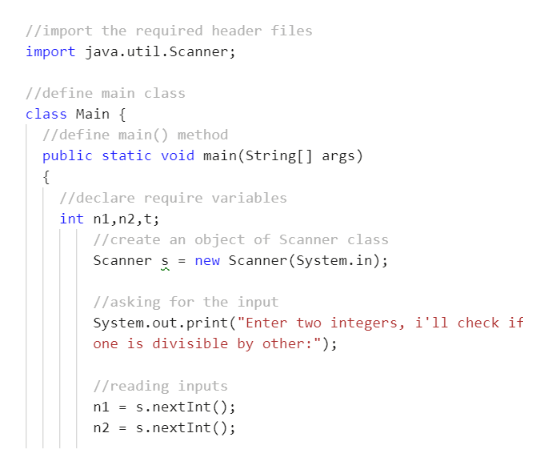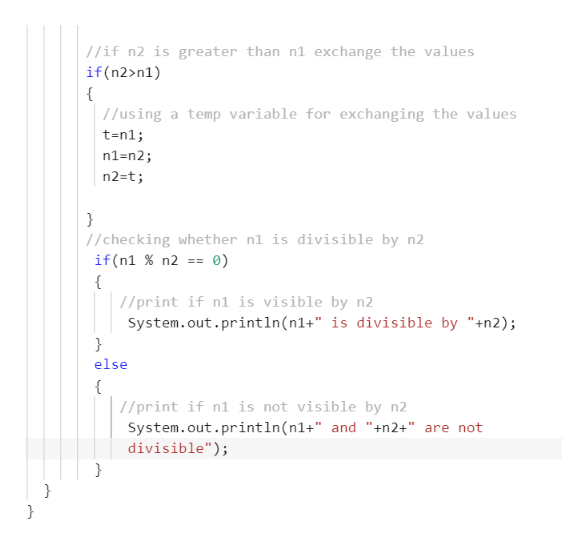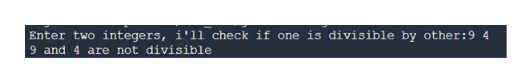PART A. Write a program Is Divisible that reads two integers from the user and determines if one is divisible bythe other. Here are several examples:Enter two integers, I'll check if one is divisible by the other: 9 49 and 4 are not divisibleEnter two integers, I'll check if one is divisible by the other: 15 515 is divisible by 5Enter two integers, I'l1 check if one is divisible by the other: 5 1515 is divisible by 5

Question

/////////////

////////////

////////////

the language used is javahelp_outlineImage TranscriptionclosePART A. Write a program Is Divisible that reads two integers from the user and determines if one is divisible by the other. Here are several examples: Enter two integers, I'll check if one is divisible by the other: 9 4 9 and 4 are not divisible Enter two integers, I'll check if one is divisible by the other: 15 5 15 is divisible by 5 Enter two integers, I'l1 check if one is divisible by the other: 5 15 15 is divisible by 5 fullscreen
Step 1

Program code #1:help_outlineImage Transcriptionclose//import the required header files import java.util.Scanner; //define main class class Main //define main () method public static void main(String[] args) //declare require variables int n1,n2,t; //create an object of Scanner class new Scanner(System.in); Scanner s //asking for the input System.out.print("Enter two integers, i'll check if one is divisible by other:"); //reading inputs s. nextInt () s.nextInt() n1 = n2 fullscreen
Step 2

Program code #2:help_outlineImage Transcriptionclose//if n2 is greater than n1 exchange the values if(n2>n1) { /using a temp variable for exchanging the values t-n1 n1-n2; n2=t; } //checking whether n1 is divisible by n2 if (n1 % n2 { //print if n1 is visible by n2 System.out.println(n1+" is divisible by "+n2); } else { //print if n1 is not visible by n2 System.out.println(n1+" and "+n2+" are not divisible"); } } } fullscreen
Step 3

Output #1:

...help_outlineImage TranscriptioncloseEnter two integers, i'll check if one is divisible by other:9 4 9 and 4 are not divisible fullscreen

Want to see the full answer?

See Solution

Want to see this answer and more?

Our solutions are written by experts, many with advanced degrees, and available 24/7

See Solution
Tagged in

Programing Language